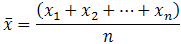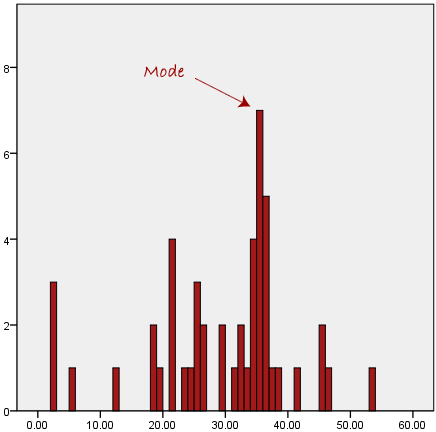# Measures of Central Tendency: Mean, Median, and Mode

A measure of central tendency is a summary statistic that represents the center point or typical value of a dataset. These measures indicate where most values in a distribution fall and are also referred to as the central location of a distribution. You can think of it as the tendency of data to cluster around a middle value. In statistics, the three most common measures of central tendency are the mean, median, and mode. Each of these measures calculates the location of the central point using a different method.

The mean, median and mode are all valid measures of central tendency, but under different conditions, some measures of central tendency become more appropriate to use than others. In the following sections, we will look at the mean, mode and median, and learn how to calculate them and under what conditions they are most appropriate to be used.

MEAN (ARITHMETIC)

The mean (or average) is the most popular and well known measure of central tendency. It can be used with both discrete and continuous data, although its use is most often with continuous data (see our Types of Variable guide for data types). The mean is equal to the sum of all the values in the data set divided by the number of values in the data set. So, if we have n values in a data set and they have values x1, x2, …, xn, the sample mean, usually denoted by  (pronounced x bar), is:This formula is usually written in a slightly different manner using the Greek capitol letter, , pronounced “sigma”, which means “sum of…”:You may have noticed that the above formula refers to the sample mean. So, why have we called it a sample mean? This is because, in statistics, samples and populations have very different meanings and these differences are very important, even if, in the case of the mean, they are calculated in the same way. To acknowledge that we are calculating the population mean and not the sample mean, we use the Greek lower case letter “mu”, denoted as µ:The mean is essentially a model of your data set. It is the value that is most common. You will notice, however, that the mean is not often one of the actual values that you have observed in your data set. However, one of its important properties is that it minimizes error in the prediction of any one value in your data set. That is, it is the value that produces the lowest amount of error from all other values in the data set.

An important property of the mean is that it includes every value in your data set as part of the calculation. In addition, the mean is the only measure of central tendency where the sum of the deviations of each value from the mean is always zero.

MEDIAN

The median is the middle score for a set of data that has been arranged in order of magnitude. The median is less affected by outliers and skewed data. In order to calculate the median, suppose we have the data below:

 65 55 89 56 35 14 56 55 87 45 92

We first need to rearrange that data into order of magnitude (smallest first):

 14 35 45 55 55 56 56 65 87 89 92

Our median mark is the middle mark – in this case, 56 (highlighted in bold). It is the middle mark because there are 5 scores before it and 5 scores after it. This works fine when you have an odd number of scores, but what happens when you have an even number of scores? What if you had only 10 scores? Well, you simply have to take the middle two scores and average the result. So, if we look at the example below:

 65 55 89 56 35 14 56 55 87 45

We again rearrange that data into order of magnitude (smallest first):

 14 35 45 55 55 56 56 65 87 89

Only now we have to take the 5th and 6th score in our data set and average them to get a median of 55.5.

MODE

The mode is the most frequent score in our data set. On a histogram it represents the highest bar in a bar chart or histogram. You can, therefore, sometimes consider the mode as being the most popular option. An example of a mode is presented below:## 5 thoughts on “Measures of Central Tendency: Mean, Median, and Mode”

error: Content is protected !!